Successfully reported this slideshow.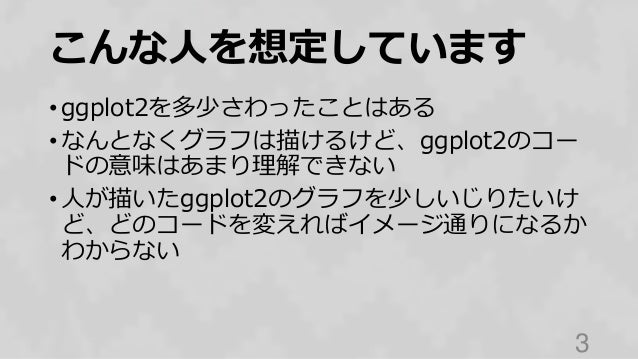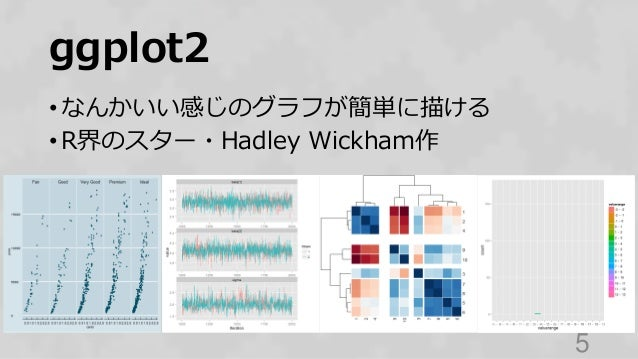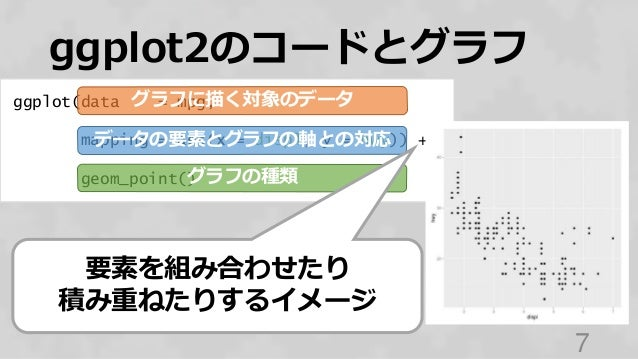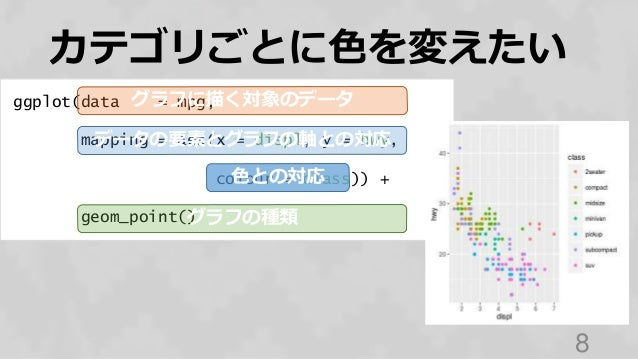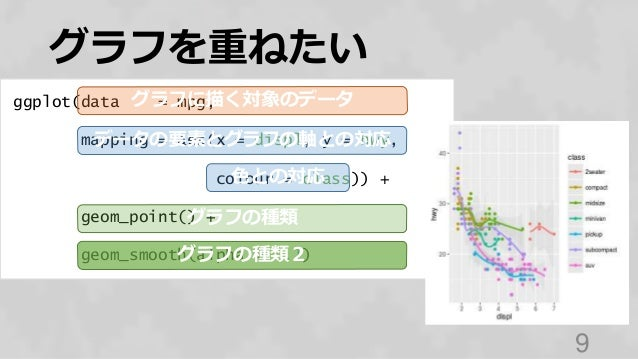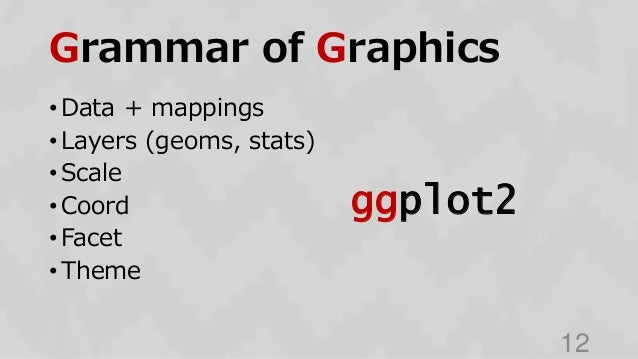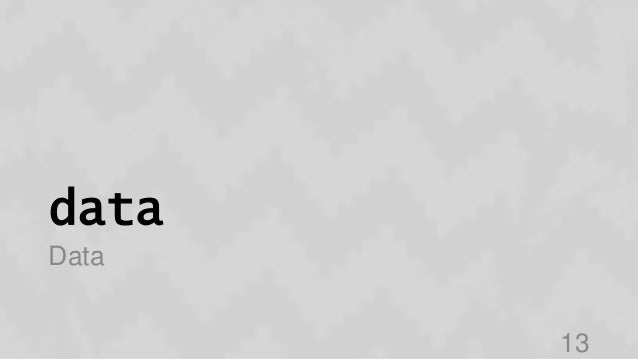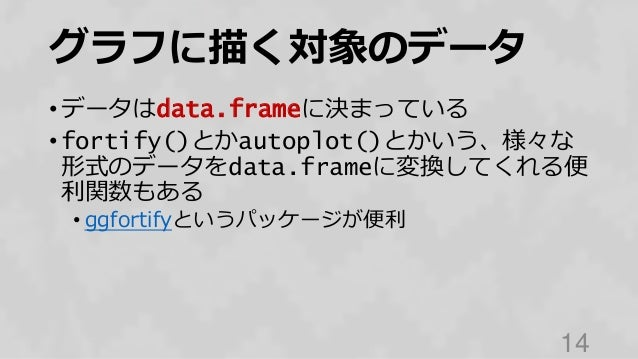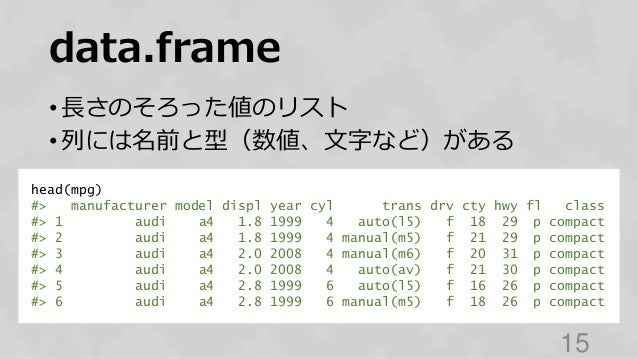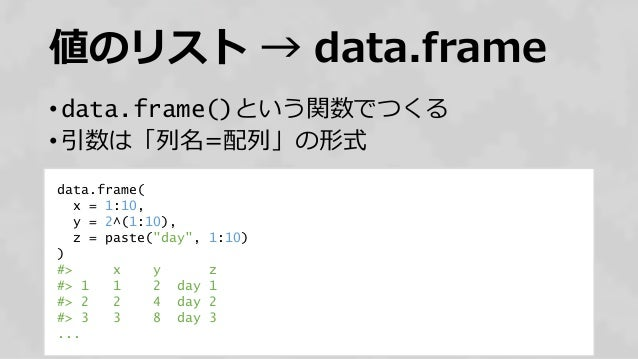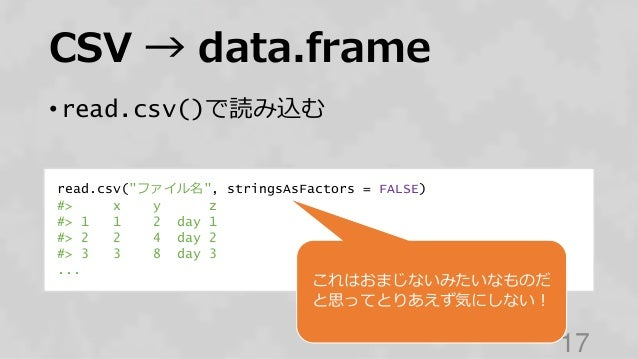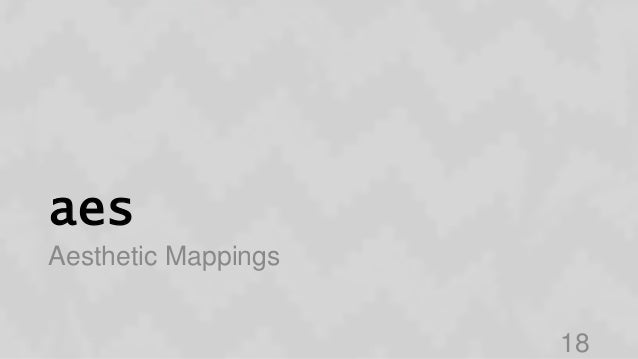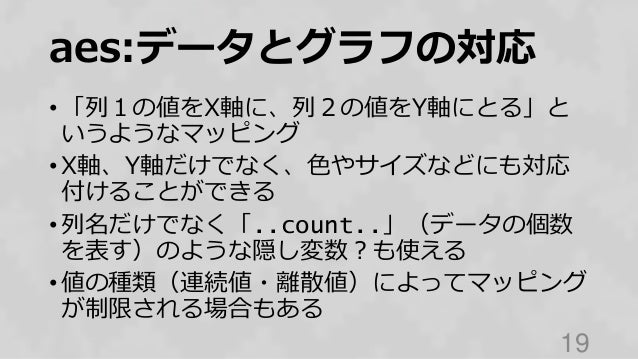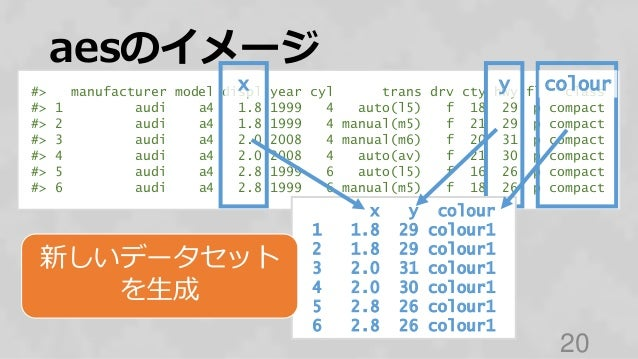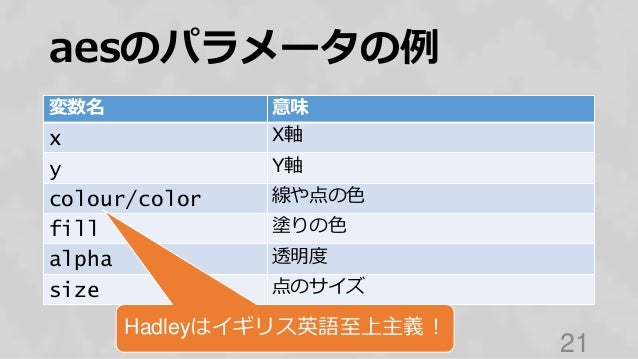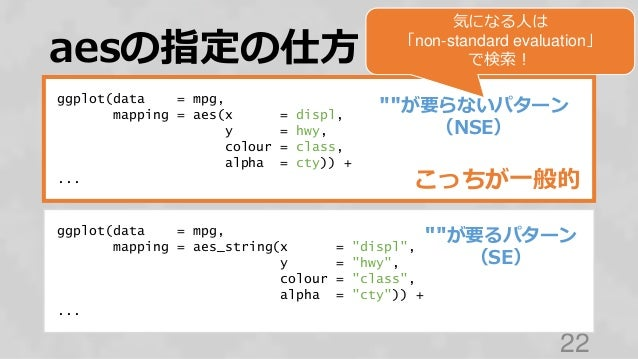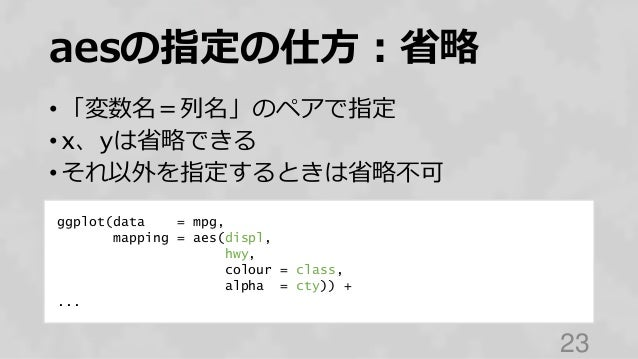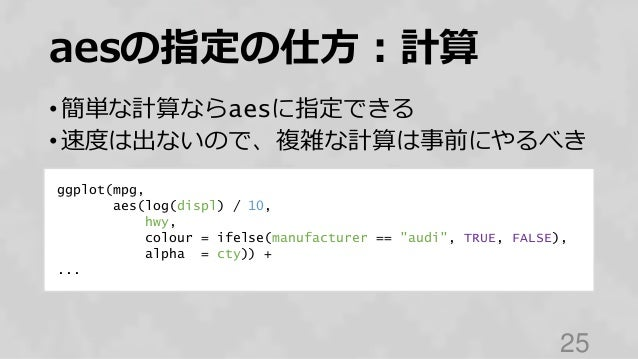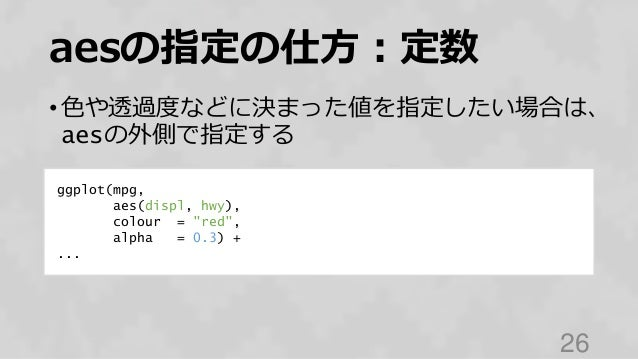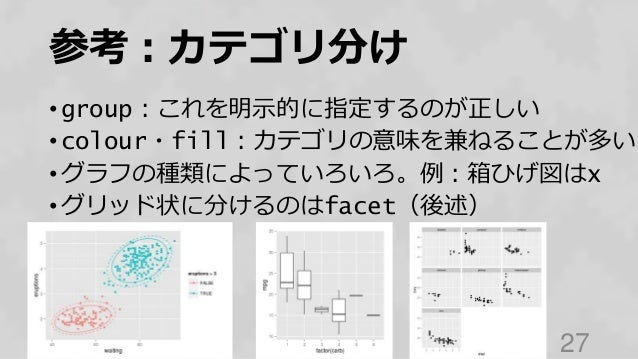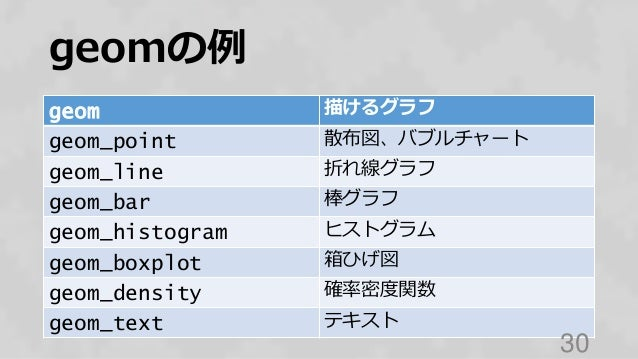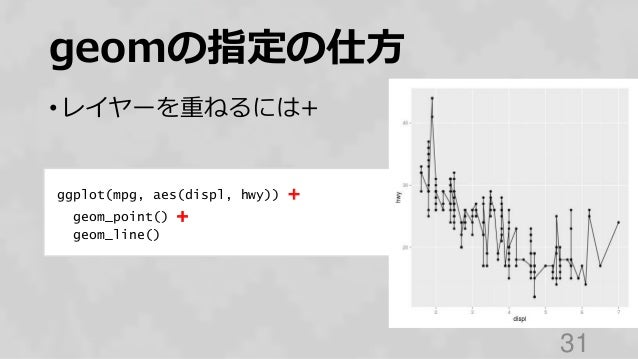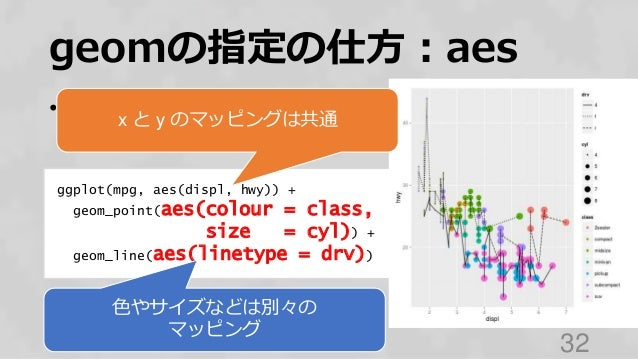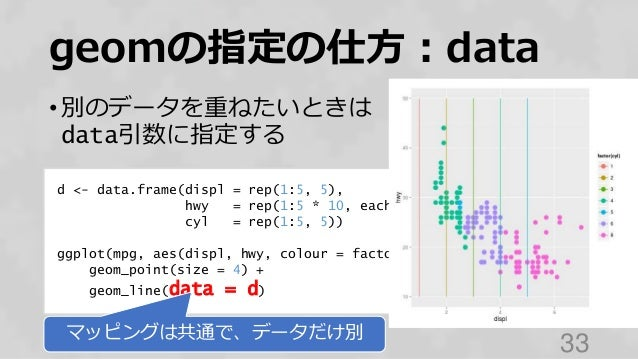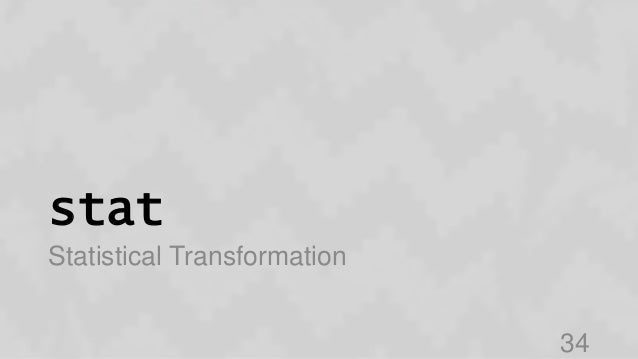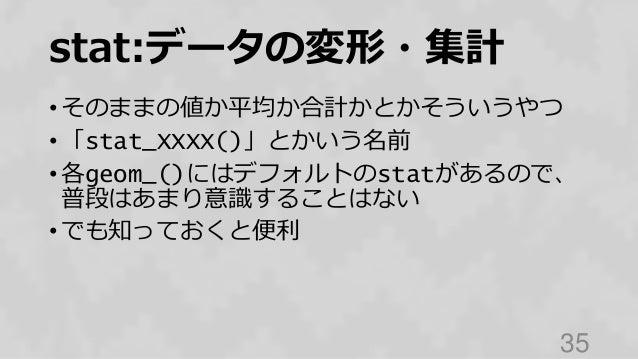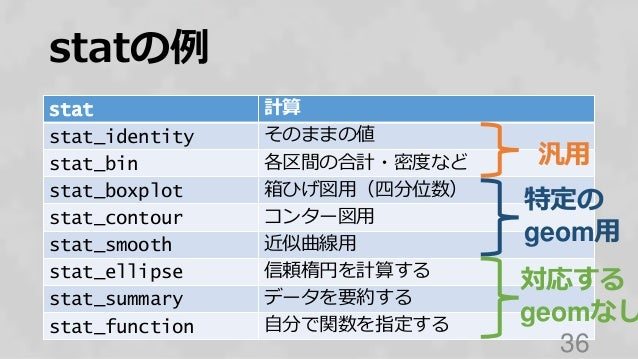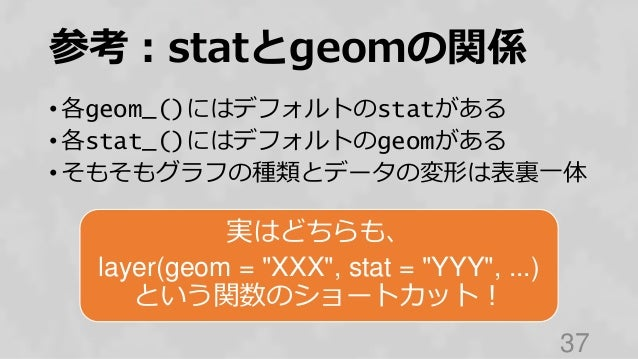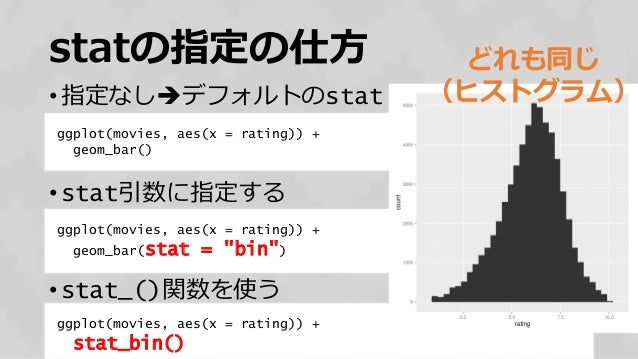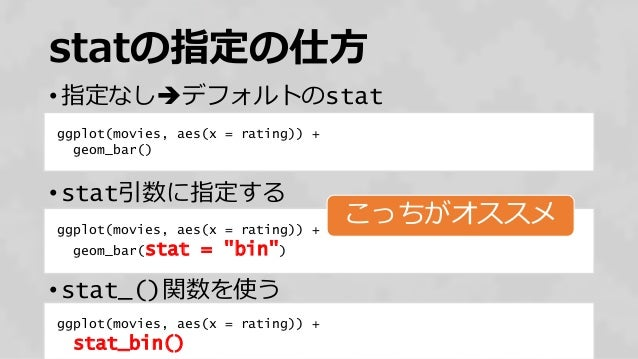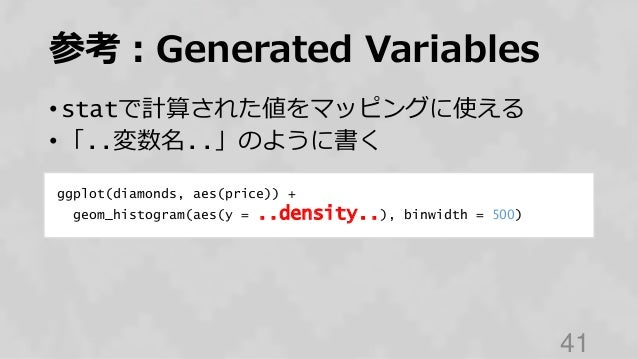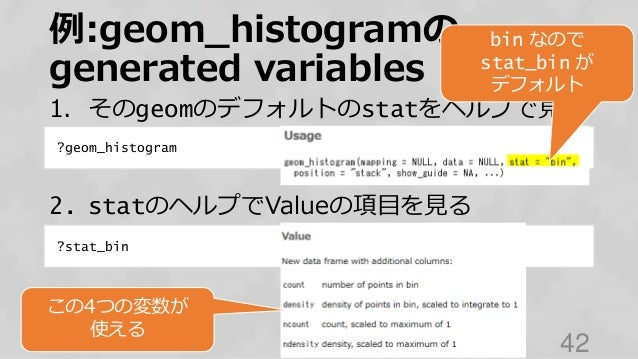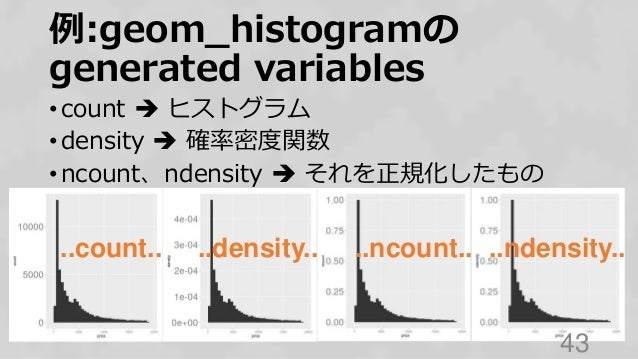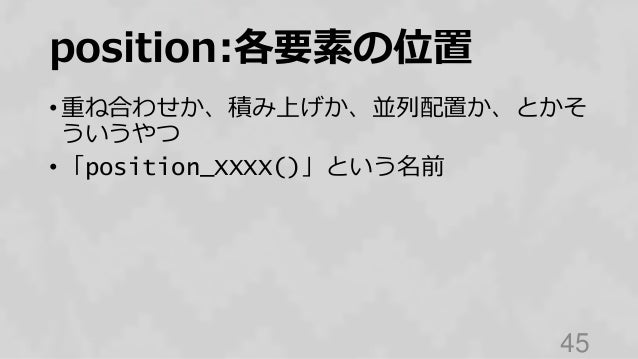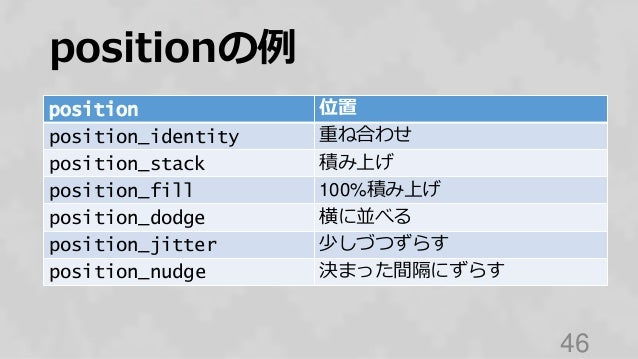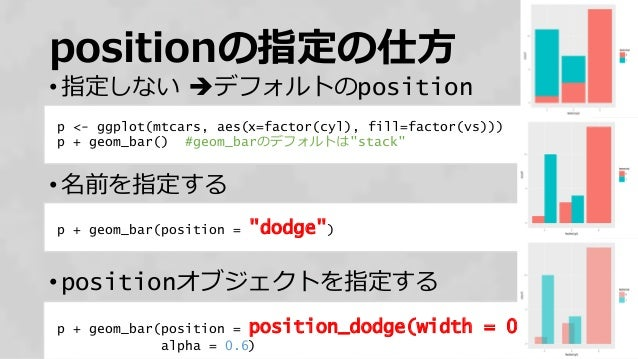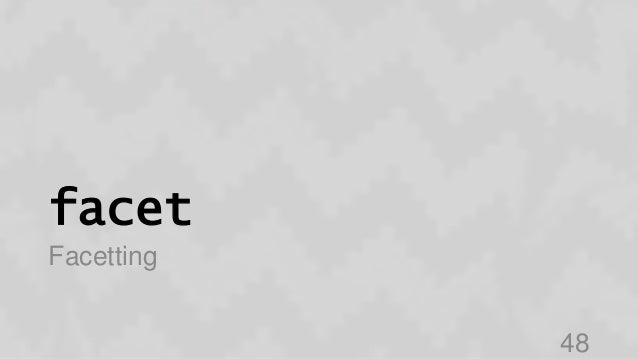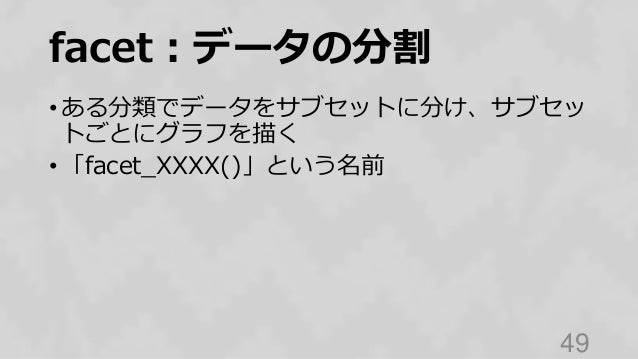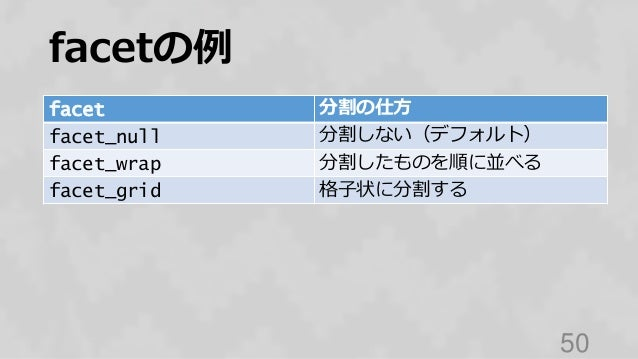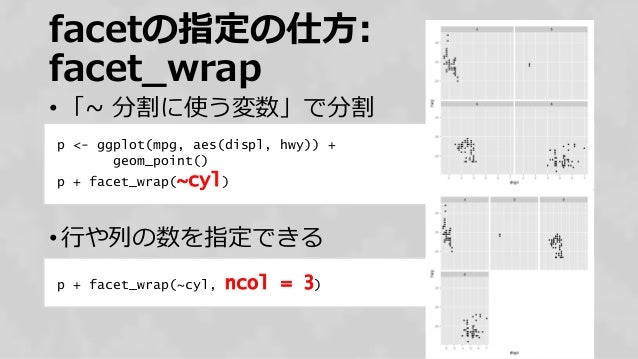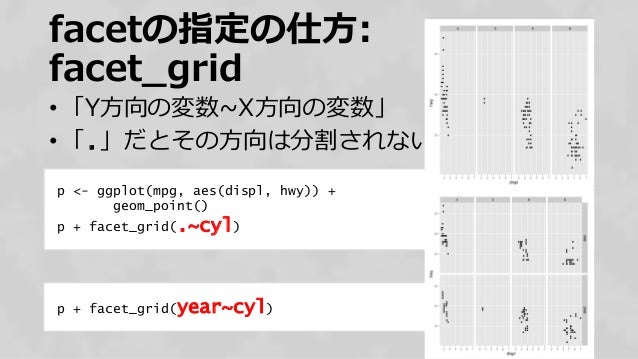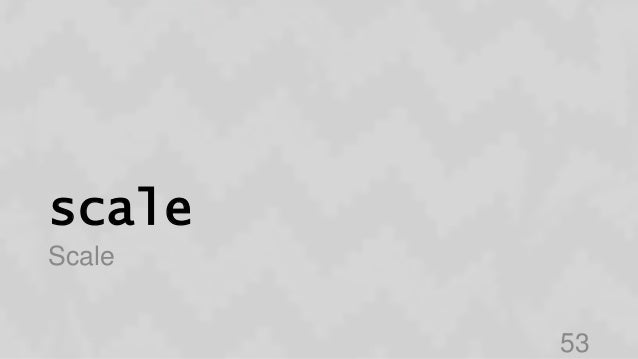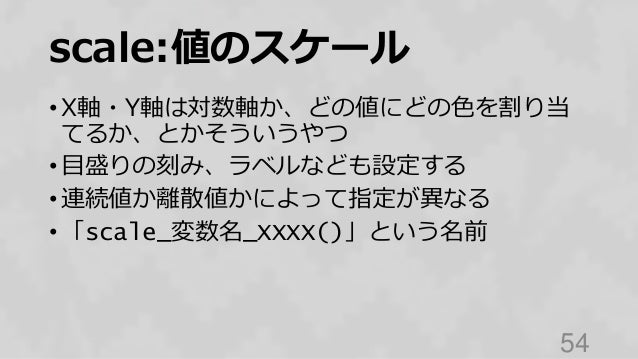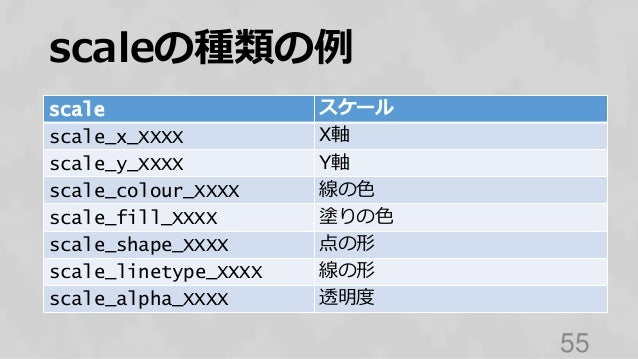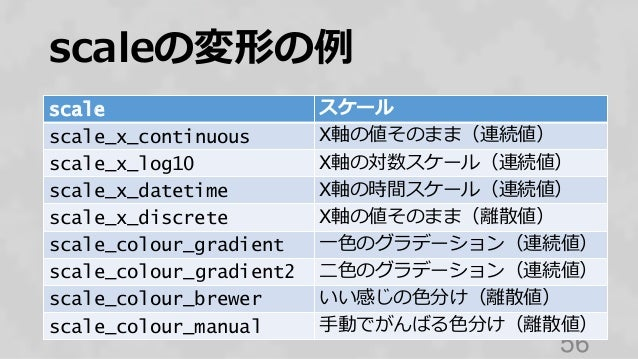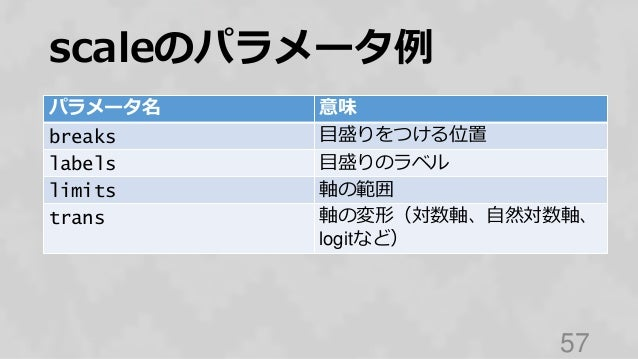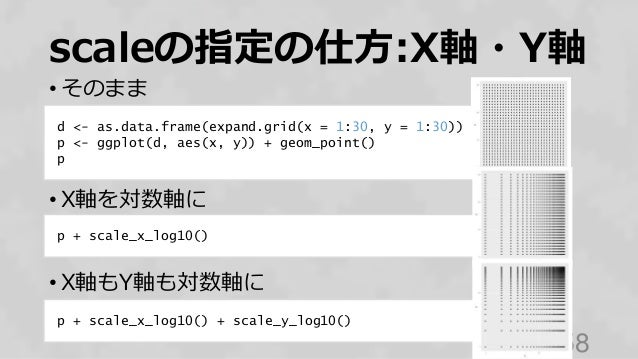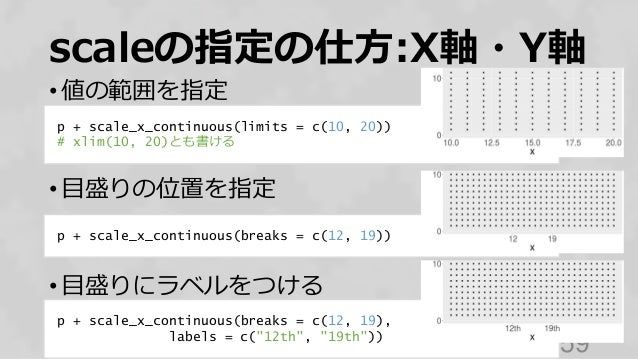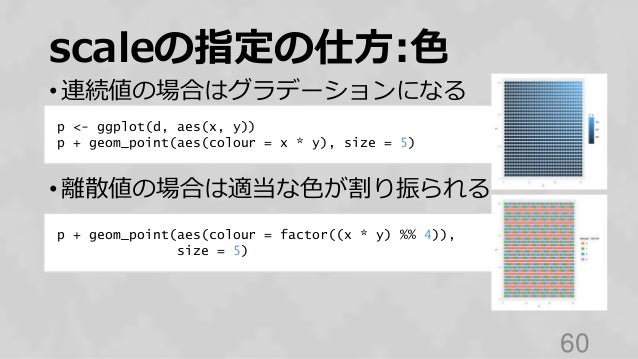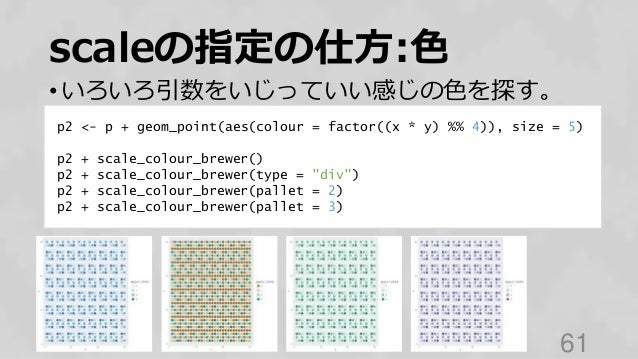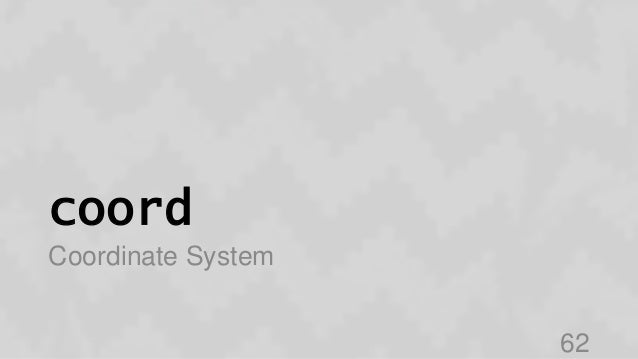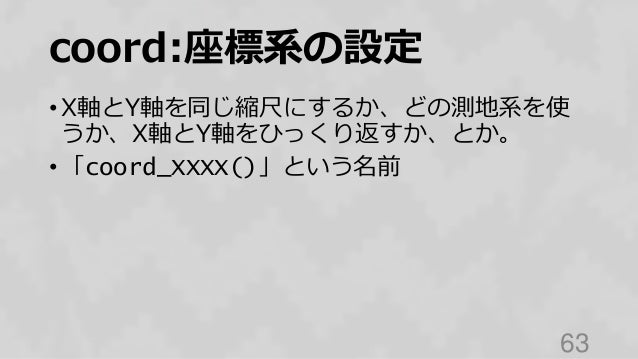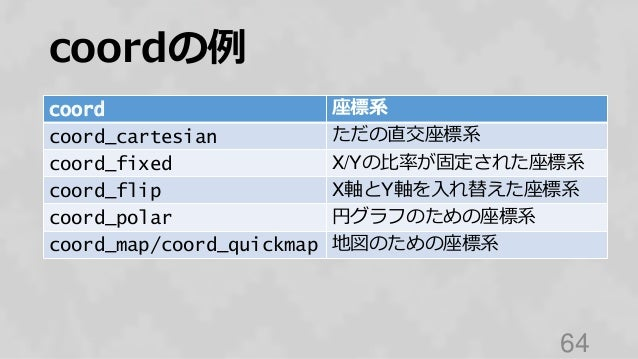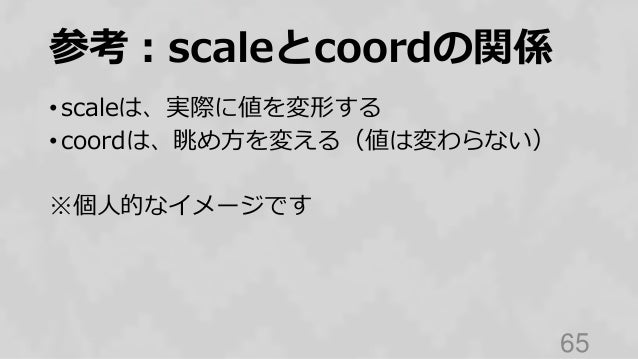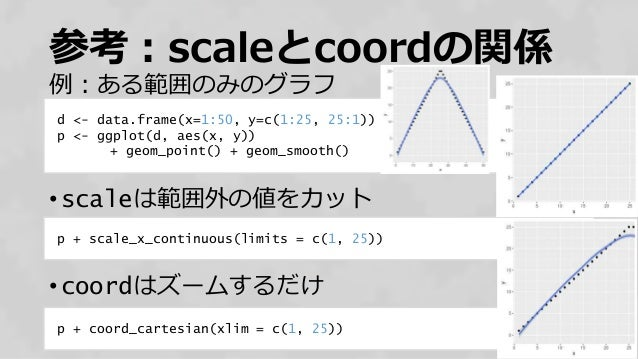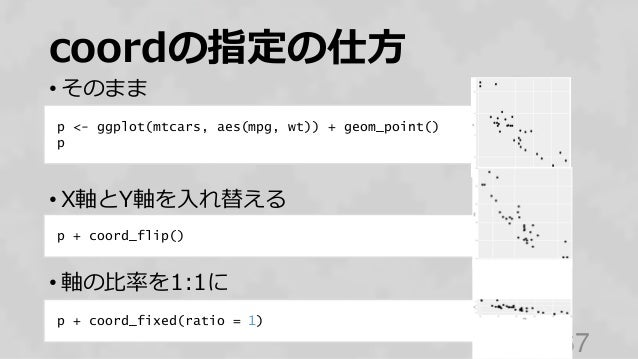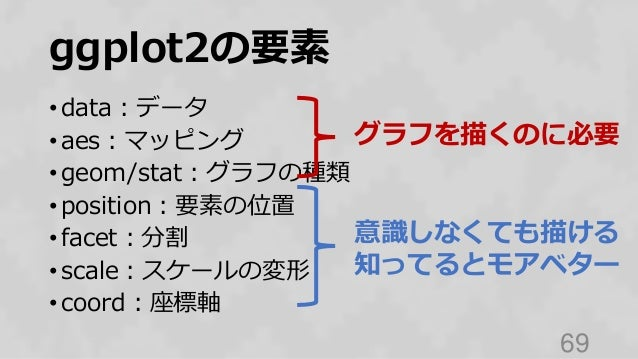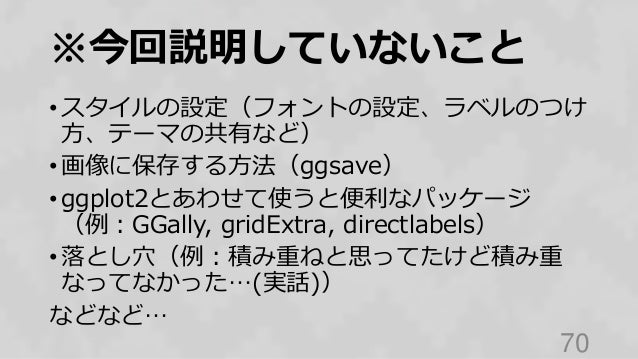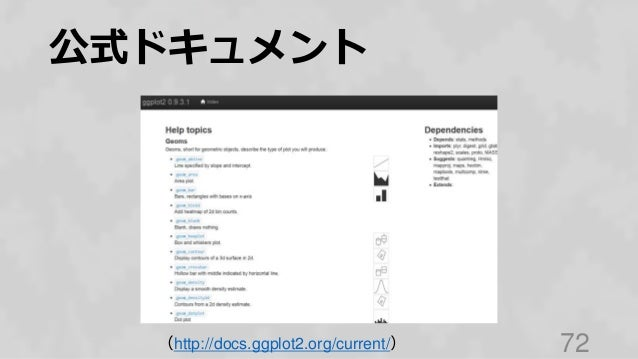Upcoming SlideShare
×
• Full Name
Comment goes here.

Are you sure you want to Yes No• Be the first to comment

### ggplot2再入門（2015年バージョン）

1. 1. ggplot2再入門 2015/09/24 Rと統計の勉強会 @yutannihilation 1
2. 2. 対象 2
3. 3. こんな人を想定しています • ggplot2を多少さわったことはある • なんとなくグラフは描けるけど、ggplot2のコー ドの意味はあまり理解できない • 人が描いたggplot2のグラフを少しいじりたいけ ど、どのコードを変えればイメージ通りになるか わからない 3
4. 4. ggplot2とは 4
5. 5. ggplot2 • なんかいい感じのグラフが簡単に描ける • R界のスター・Hadley Wickham作 5
6. 6. いい感じのグラフとは 6
7. 7. ggplot(data = mpg, mapping = aes(x = displ, y = hwy)) + geom_point() ggplot2のコードとグラフ 7 グラフに描く対象のデータ データの要素とグラフの軸との対応 グラフの種類 要素を組み合わせたり 積み重ねたりするイメージ
8. 8. ggplot(data = mpg, mapping = aes(x = displ, y = hwy, colour = class)) + geom_point() カテゴリごとに色を変えたい 8 グラフに描く対象のデータ データの要素とグラフの軸との対応 グラフの種類 色との対応
9. 9. ggplot(data = mpg, mapping = aes(x = displ, y = hwy, colour = class)) + geom_point() + geom_smooth(alpha = 0.1) グラフを重ねたい 9 グラフに描く対象のデータ データの要素とグラフの軸との対応 グラフの種類 色との対応 グラフの種類２
10. 10. 何がいい感じなのか グラフの要素がいい感じに分割されている。レゴブ ロック的な。 • 設定を組み替えたり変えたりしやすい → 探索的なデータ分析に便利 → 複雑なグラフを描くときも見通しが立つ • でもレゴと同じく踏むと痛いこともある…。独特！ 10
11. 11. グラフの要素とは 11
12. 12. Grammar of Graphics • Data + mappings • Layers (geoms, stats) • Scale • Coord • Facet • Theme 12 ggplot2
13. 13. data Data 13
14. 14. グラフに描く対象のデータ • データはdata.frameに決まっている • fortify()とかautoplot()とかいう、様々な 形式のデータをdata.frameに変換してくれる便 利関数もある • ggfortifyというパッケージが便利 14
15. 15. data.frame • 長さのそろった値のリスト • 列には名前と型（数値、文字など）がある 15 head(mpg) #> manufacturer model displ year cyl trans drv cty hwy fl class #> 1 audi a4 1.8 1999 4 auto(l5) f 18 29 p compact #> 2 audi a4 1.8 1999 4 manual(m5) f 21 29 p compact #> 3 audi a4 2.0 2008 4 manual(m6) f 20 31 p compact #> 4 audi a4 2.0 2008 4 auto(av) f 21 30 p compact #> 5 audi a4 2.8 1999 6 auto(l5) f 16 26 p compact #> 6 audi a4 2.8 1999 6 manual(m5) f 18 26 p compact
16. 16. 値のリスト → data.frame • data.frame()という関数でつくる • 引数は「列名=配列」の形式 16 data.frame( x = 1:10, y = 2^(1:10), z = paste("day", 1:10) ) #> x y z #> 1 1 2 day 1 #> 2 2 4 day 2 #> 3 3 8 day 3 ...
17. 17. CSV → data.frame • read.csv()で読み込む 17 read.csv("ファイル名", stringsAsFactors = FALSE) #> x y z #> 1 1 2 day 1 #> 2 2 4 day 2 #> 3 3 8 day 3 ... これはおまじないみたいなものだ と思ってとりあえず気にしない！
18. 18. aes Aesthetic Mappings 18
19. 19. aes:データとグラフの対応 • 「列１の値をX軸に、列２の値をY軸にとる」と いうようなマッピング • X軸、Y軸だけでなく、色やサイズなどにも対応 付けることができる • 列名だけでなく「..count..」（データの個数 を表す）のような隠し変数？も使える • 値の種類（連続値・離散値）によってマッピング が制限される場合もある 19
20. 20. aesのイメージ 20 #> manufacturer model displ year cyl trans drv cty hwy fl class #> 1 audi a4 1.8 1999 4 auto(l5) f 18 29 p compact #> 2 audi a4 1.8 1999 4 manual(m5) f 21 29 p compact #> 3 audi a4 2.0 2008 4 manual(m6) f 20 31 p compact #> 4 audi a4 2.0 2008 4 auto(av) f 21 30 p compact #> 5 audi a4 2.8 1999 6 auto(l5) f 16 26 p compact #> 6 audi a4 2.8 1999 6 manual(m5) f 18 26 p compact x y colour x y colour 1 1.8 29 colour1 2 1.8 29 colour1 3 2.0 31 colour1 4 2.0 30 colour1 5 2.8 26 colour1 6 2.8 26 colour1 新しいデータセット を生成
21. 21. aesのパラメータの例 変数名 意味 x X軸 y Y軸 colour/color 線や点の色 fill 塗りの色 alpha 透明度 size 点のサイズ 21 Hadleyはイギリス英語至上主義！
22. 22. aesの指定の仕方 22 ggplot(data = mpg, mapping = aes_string(x = "displ", y = "hwy", colour = "class", alpha = "cty")) + ... ggplot(data = mpg, mapping = aes(x = displ, y = hwy, colour = class, alpha = cty)) + ... ""が要らないパターン （NSE） ""が要るパターン （SE） こっちが一般的 気になる人は 「non-standard evaluation」 で検索！
23. 23. aesの指定の仕方：省略 • 「変数名＝列名」のペアで指定 • x、yは省略できる • それ以外を指定するときは省略不可 23 ggplot(data = mpg, mapping = aes(displ, hwy, colour = class, alpha = cty)) + ...
24. 24. aesの指定の仕方：省略 • ついでにいうと、dataとかmappingも省略可 • この書き方が一般的なので慣れましょう 24 ggplot(mpg, aes(displ, hwy, colour = class, alpha = cty)) + ...
25. 25. aesの指定の仕方：計算 • 簡単な計算ならaesに指定できる • 速度は出ないので、複雑な計算は事前にやるべき 25 ggplot(mpg, aes(log(displ) / 10, hwy, colour = ifelse(manufacturer == "audi", TRUE, FALSE), alpha = cty)) + ...
26. 26. aesの指定の仕方：定数 • 色や透過度などに決まった値を指定したい場合は、 aesの外側で指定する 26 ggplot(mpg, aes(displ, hwy), colour = "red", alpha = 0.3) + ...
27. 27. 参考：カテゴリ分け • group：これを明示的に指定するのが正しい • colour・fill：カテゴリの意味を兼ねることが多い • グラフの種類によっていろいろ。例：箱ひげ図はx • グリッド状に分けるのはfacet（後述） 27
28. 28. geom Geometric Objects 28
29. 29. geom:グラフの種類 • 棒グラフとか散布図とかそういうやつ • 「geom_XXXX()」という名前 29
30. 30. geomの例 geom 描けるグラフ geom_point 散布図、バブルチャート geom_line 折れ線グラフ geom_bar 棒グラフ geom_histogram ヒストグラム geom_boxplot 箱ひげ図 geom_density 確率密度関数 geom_text テキスト 30
31. 31. geomの指定の仕方 • レイヤーを重ねるには+ 31 ggplot(mpg, aes(displ, hwy)) + geom_point() + geom_line()
32. 32. geomの指定の仕方：aes • 別のaesを使いたいときは それぞれに指定する 32 ggplot(mpg, aes(displ, hwy)) + geom_point(aes(colour = class, size = cyl)) + geom_line(aes(linetype = drv)) x と y のマッピングは共通 色やサイズなどは別々の マッピング
33. 33. geomの指定の仕方：data • 別のデータを重ねたいときは data引数に指定する 33 d <- data.frame(displ = rep(1:5, 5), hwy = rep(1:5 * 10, each = 5), cyl = rep(1:5, 5)) ggplot(mpg, aes(displ, hwy, colour = factor(cyl))) + geom_point(size = 4) + geom_line(data = d) マッピングは共通で、データだけ別
34. 34. stat Statistical Transformation 34
35. 35. stat:データの変形・集計 • そのままの値か平均か合計かとかそういうやつ • 「stat_XXXX()」とかいう名前 • 各geom_()にはデフォルトのstatがあるので、 普段はあまり意識することはない • でも知っておくと便利 35
36. 36. statの例 stat 計算 stat_identity そのままの値 stat_bin 各区間の合計・密度など stat_boxplot 箱ひげ図用（四分位数） stat_contour コンター図用 stat_smooth 近似曲線用 stat_ellipse 信頼楕円を計算する stat_summary データを要約する stat_function 自分で関数を指定する 36 汎用 特定の geom用 対応する geomなし
37. 37. 参考：statとgeomの関係 • 各geom_()にはデフォルトのstatがある • 各stat_()にはデフォルトのgeomがある • そもそもグラフの種類とデータの変形は表裏一体 37 実はどちらも、 layer(geom = "XXX", stat = "YYY", ...) という関数のショートカット！
38. 38. 参考：statとgeomの関係 • 作者の「わかりにくくてごめん！」という懺悔： Unfortunately, due to any early design mistake I called these either stat_() or geom_(). A better decision would have been to call them layer_() functions: that's a more accurate description because every layer involves a a stat and a geom. （出典：https://github.com/hadley/ggplot2/blob/master/vignettes/extending- ggplot2.Rmd） 38
39. 39. statの指定の仕方 • 指定なしデフォルトのstat • stat引数に指定する • stat_()関数を使う 39 ggplot(movies, aes(x = rating)) + stat_bin() ggplot(movies, aes(x = rating)) + geom_bar() ggplot(movies, aes(x = rating)) + geom_bar(stat = "bin") どれも同じ （ヒストグラム）
40. 40. statの指定の仕方 • 指定なしデフォルトのstat • stat引数に指定する • stat_()関数を使う 40 ggplot(movies, aes(x = rating)) + stat_bin() ggplot(movies, aes(x = rating)) + geom_bar() ggplot(movies, aes(x = rating)) + geom_bar(stat = "bin") こっちがオススメ
41. 41. 参考：Generated Variables • statで計算された値をマッピングに使える • 「..変数名..」のように書く 41 ggplot(diamonds, aes(price)) + geom_histogram(aes(y = ..density..), binwidth = 500)
42. 42. 例:geom_histogramの generated variables 1. そのgeomのデフォルトのstatをヘルプで見る 2. statのヘルプでValueの項目を見る 42 ?geom_histogram ?stat_bin bin なので stat_bin が デフォルト この4つの変数が 使える
43. 43. • count  ヒストグラム • density  確率密度関数 • ncount、ndensity  それを正規化したもの 43 ..count.. ..density.. ..ncount.. ..ndensity.. 例:geom_histogramの generated variables
44. 44. position Position Adjustments 44
45. 45. position:各要素の位置 • 重ね合わせか、積み上げか、並列配置か、とかそ ういうやつ • 「position_XXXX()」という名前 45
46. 46. positionの例 46 position 位置 position_identity 重ね合わせ position_stack 積み上げ position_fill 100%積み上げ position_dodge 横に並べる position_jitter 少しづつずらす position_nudge 決まった間隔にずらす
47. 47. positionの指定の仕方 • 指定しない デフォルトのposition • 名前を指定する • positionオブジェクトを指定する 47 p <- ggplot(mtcars, aes(x=factor(cyl), fill=factor(vs))) p + geom_bar() #geom_barのデフォルトは"stack" p + geom_bar(position = "dodge") p + geom_bar(position = position_dodge(width = 0.5), alpha = 0.6)
48. 48. facet Facetting 48
49. 49. facet：データの分割 • ある分類でデータをサブセットに分け、サブセッ トごとにグラフを描く • 「facet_XXXX()」という名前 49
50. 50. facetの例 50 facet 分割の仕方 facet_null 分割しない（デフォルト） facet_wrap 分割したものを順に並べる facet_grid 格子状に分割する
51. 51. facetの指定の仕方: facet_wrap • 「~ 分割に使う変数」で分割 • 行や列の数を指定できる 51 p <- ggplot(mpg, aes(displ, hwy)) + geom_point() p + facet_wrap(~cyl) p + facet_wrap(~cyl, ncol = 3)
52. 52. facetの指定の仕方: facet_grid • 「Y方向の変数~X方向の変数」 • 「.」だとその方向は分割されない 52 p <- ggplot(mpg, aes(displ, hwy)) + geom_point() p + facet_grid(.~cyl) p + facet_grid(year~cyl)
53. 53. scale Scale 53
54. 54. scale:値のスケール • X軸・Y軸は対数軸か、どの値にどの色を割り当 てるか、とかそういうやつ • 目盛りの刻み、ラベルなども設定する • 連続値か離散値かによって指定が異なる • 「scale_変数名_XXXX()」という名前 54
55. 55. scaleの種類の例 55 scale スケール scale_x_XXXX X軸 scale_y_XXXX Y軸 scale_colour_XXXX 線の色 scale_fill_XXXX 塗りの色 scale_shape_XXXX 点の形 scale_linetype_XXXX 線の形 scale_alpha_XXXX 透明度
56. 56. scaleの変形の例 56 scale スケール scale_x_continuous X軸の値そのまま（連続値） scale_x_log10 X軸の対数スケール（連続値） scale_x_datetime X軸の時間スケール（連続値） scale_x_discrete X軸の値そのまま（離散値） scale_colour_gradient 一色のグラデーション（連続値） scale_colour_gradient2 二色のグラデーション（連続値） scale_colour_brewer いい感じの色分け（離散値） scale_colour_manual 手動でがんばる色分け（離散値）
57. 57. scaleのパラメータ例 57 パラメータ名 意味 breaks 目盛りをつける位置 labels 目盛りのラベル limits 軸の範囲 trans 軸の変形（対数軸、自然対数軸、 logitなど）
58. 58. scaleの指定の仕方:X軸・Y軸 • そのまま • X軸を対数軸に • X軸もY軸も対数軸に 58 d <- as.data.frame(expand.grid(x = 1:30, y = 1:30)) p <- ggplot(d, aes(x, y)) + geom_point() p p + scale_x_log10() p + scale_x_log10() + scale_y_log10()
59. 59. scaleの指定の仕方:X軸・Y軸 • 値の範囲を指定 • 目盛りの位置を指定 • 目盛りにラベルをつける 59 p + scale_x_continuous(limits = c(10, 20)) # xlim(10, 20)とも書ける p + scale_x_continuous(breaks = c(12, 19)) p + scale_x_continuous(breaks = c(12, 19), labels = c("12th", "19th"))
60. 60. scaleの指定の仕方:色 • 連続値の場合はグラデーションになる • 離散値の場合は適当な色が割り振られる 60 p <- ggplot(d, aes(x, y)) p + geom_point(aes(colour = x * y), size = 5) p + geom_point(aes(colour = factor((x * y) %% 4)), size = 5)
61. 61. scaleの指定の仕方:色 • いろいろ引数をいじっていい感じの色を探す。 61 p2 <- p + geom_point(aes(colour = factor((x * y) %% 4)), size = 5) p2 + scale_colour_brewer() p2 + scale_colour_brewer(type = "div") p2 + scale_colour_brewer(pallet = 2) p2 + scale_colour_brewer(pallet = 3)
62. 62. coord Coordinate System 62
63. 63. coord:座標系の設定 • X軸とY軸を同じ縮尺にするか、どの測地系を使 うか、X軸とY軸をひっくり返すか、とか。 • 「coord_XXXX()」という名前 63
64. 64. coordの例 64 coord 座標系 coord_cartesian ただの直交座標系 coord_fixed X/Yの比率が固定された座標系 coord_flip X軸とY軸を入れ替えた座標系 coord_polar 円グラフのための座標系 coord_map/coord_quickmap 地図のための座標系
65. 65. 参考：scaleとcoordの関係 • scaleは、実際に値を変形する • coordは、眺め方を変える（値は変わらない） ※個人的なイメージです 65
66. 66. 参考：scaleとcoordの関係 66 p + coord_cartesian(xlim = c(1, 25)) d <- data.frame(x=1:50, y=c(1:25, 25:1)) p <- ggplot(d, aes(x, y)) + geom_point() + geom_smooth() p + scale_x_continuous(limits = c(1, 25)) 例：ある範囲のみのグラフ • scaleは範囲外の値をカット • coordはズームするだけ
67. 67. coordの指定の仕方 • そのまま • X軸とY軸を入れ替える • 軸の比率を1:1に 67 p <- ggplot(mtcars, aes(mpg, wt)) + geom_point() p p + coord_flip() p + coord_fixed(ratio = 1)
68. 68. まとめ 68
69. 69. ggplot2の要素 • data：データ • aes：マッピング • geom/stat：グラフの種類 • position：要素の位置 • facet：分割 • scale：スケールの変形 • coord：座標軸 69 グラフを描くのに必要 意識しなくても描ける 知ってるとモアベター
70. 70. ※今回説明していないこと • スタイルの設定（フォントの設定、ラベルのつけ 方、テーマの共有など） • 画像に保存する方法（ggsave） • ggplot2とあわせて使うと便利なパッケージ （例：GGally, gridExtra, directlabels） • 落とし穴（例：積み重ねと思ってたけど積み重 なってなかった…(実話)） などなど… 70
71. 71. 分からないときは 71
72. 72. 公式ドキュメント 72（http://docs.ggplot2.org/current/）
75. 75. ggplot2逆引き 75(https://yutannihilation.github.io/ggplot2-gyakubiki/)
76. 76. r-wakalang （Rについて気軽に質問できるチャット。オススメ！） 76（詳しくは、http://www.slideshare.net/teramonagi/ss-52463319）
77. 77. 77 Enjoy!

### Be the first to comment

•#### yasuhiroazuma9

Sep. 27, 2018
•#### vym0523

Dec. 7, 2018
•#### ChigayaEnju

Dec. 22, 2018
•#### DaisukeT1

Feb. 7, 2019
•#### tomoyukinoguchi

Apr. 2, 2019
•#### ssuser81afbb

Apr. 14, 2019
•#### ssuser3e23de

Apr. 23, 2019
•#### ssuser61539f

May. 26, 2019
•#### AkiraIshii11

Jul. 9, 2019
•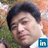#### HidekiAbe

Aug. 29, 2019
•#### yasu2015

Sep. 4, 2019
•#### OkunoTakuya

Oct. 23, 2019
•#### NaokoHirakawa

Nov. 27, 2019
•#### KosukeYamazaki2

Dec. 21, 2019
•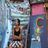#### masafumi928

Feb. 16, 2020
•#### ssuserb545b0

Mar. 5, 2020
•#### YuseiKawai

Apr. 2, 2020
•Aug. 8, 2020
•#### etzel1194

Aug. 9, 2020
•Mar. 4, 2021

Total views

59,619

On Slideshare

0

From embeds

0

Number of embeds

35,567

0

Shares

0